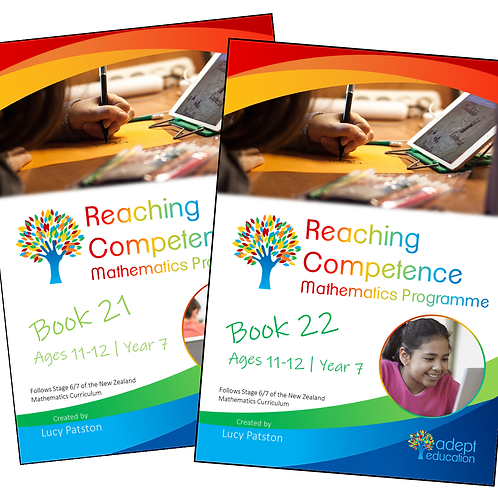`The books cover the transition from Stage 6 to Stage 7 (Year 7). Book 21 - 104 pages (57 worksheets)Book 22 - 104 pages (62 worksheets) Get these activity books in PDF format immediately upon purchase. View the sample of Book 21 here`

# Books 21-22 e-Copy 2021 Edition

\$16.90Price
• Book 21 and 22 in PDF format. Book 23 will be added to this set in late 2021.

View the sample of Book 21 here

Books 21 to 23 cover 21 learning intentions for learners aged about 11 to 12 years old.

At this level, students are able to choose appropriately from a range of part-whole strategies to solve problems with whole numbers. They are learning to apply these strategies to the addition of decimals, related fractions and integers.

For example, 5.5 + 6.8 = 5.5 + 7 – 0.2 = 12.3

Students are learning to manipulate factors mentally to solve multiplication and division problems.

For example, instead of partitioning 5 x 12 additively as (5 x 10) + (5 x 2), students use strategies such as doubling and halving, renaming  5 x 12 as 10 x 6.

When working with fractions and ratios, students apply their knowledge of multiplication and division facts.

For example, four sixths of 30 is found by dividing 30 by 6 to find a sixth, then multiplying the result by 4 to get 20.

You will also get: